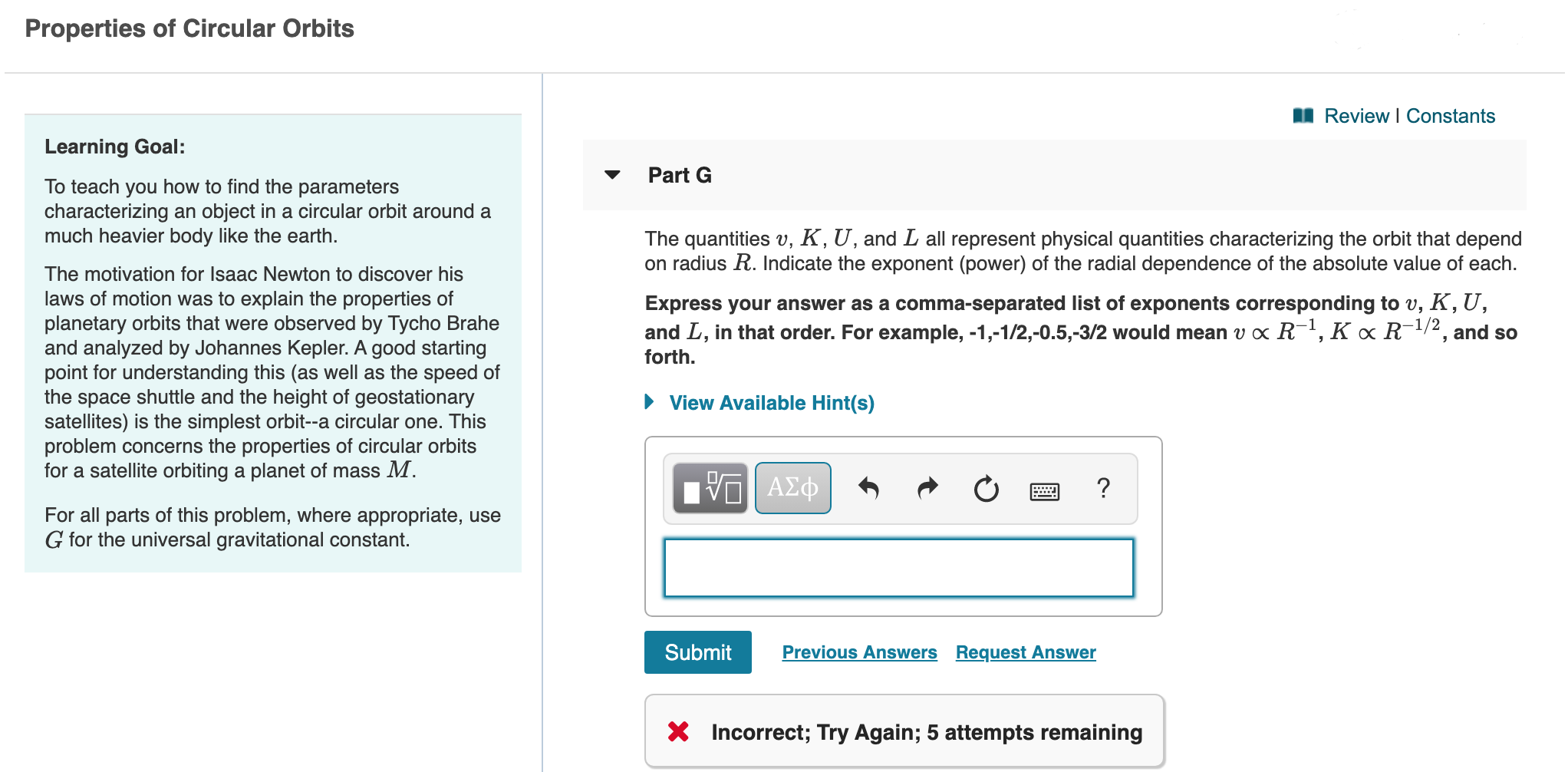# Question Properties of Circular Orbits A Review | Constants Learning Goal: Part G To teach you how to find the parameters characterizing an object in a circular orbit around a much heavier body like the earth. The quantities v, K, U, and L all represent physical quantities characterizing the orbit that depend on radius R. Indicate the exponent (power) of the radial dependence of the absolute value of each. Express your answer as a comma-separated list of exponents corresponding to v, K,U, and L, in that order. For example,-1,-1/2,-0.5,-3/2 would mean v « R-1,K « R-1/2, and so forth. The motivation for Isaac Newton to discover his laws of motion was to explain the properties of planetary orbits that were observed by Tycho Brahe and analyzed by Johannes Kepler. A good starting point for understanding this (as well as the speed of the space shuttle and the height of geostationary satellites) is the simplest orbit--a circular one. This problem concerns the properties of circular orbits for a satellite orbiting a planet of mass M. ► View Available Hint(s) Ivo A2Q * R O a ? For all parts of this problem, where appropriate, use G for the universal gravitational constant. Submit Previous Answers Request Answer X Incorrect; Try Again; 5 attempts remaining